Sie sind hier: ICP » R. Hilfer » Publikationen

# V.D Permeability Length Scales

The fact that the effective permeability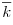has dimensions of area raises the question whether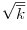has an interpretation as a length scale. The traditional answer to this question is provided by hydraulic radius theory which uses the approximate result (5.61) for the capillary tube model to postulate more generally the relation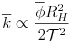(5.98)

where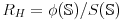is the hydraulic radius. This generalization has been modified by incorporating the formation factor to write [327, 112]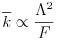(5.99)

where the length scale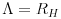is still given by the hydraulic radius, and the geometrical tortuosity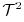was replaced by the electrical tortuosity defined as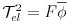. Because the length scale is still given by the hydraulic radius this theory is still faced with the objection that the hydraulic radius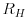contains contributions from the dead ends which do not contribute to the transport.

An alternative was proposed in [318, 43]. It postulates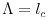where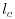is a length scale related to the breakthrough pressure in mercury injection experiments. The length scaleis well defined for network models with a broad distribution of cylindrical pores. A dynamical interpretation of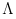was proposed in [319, 320, 328] as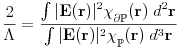(5.100)

where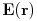is the unknown exact solution of the microscopic dielectric problem. This “electrical length” is expected to measure, somehow, the “dynamically connected pore size [319, 328, 4]. The interpretation ofwithin local porosity theory is obtained by eliminating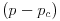between the result (5.48) for the conductivity, and equation (5.95) for the permeability. This yields in general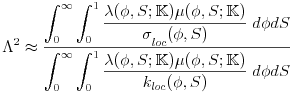(5.101)

where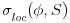and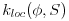are the local electrical conductivity and the local permeability. Thusinvolves macroscopic geometrical information through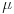and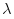and microscopic dynamical and geometrical information through the local transport coefficients. If one assumes the hydraulic radius expressions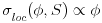and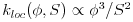locally and the expression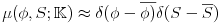valid for large measurment cells, then it follows that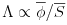becomes the local hydraulic radius . This expression is no longer proportional to the total internal surface but only to the average local internal surface, and thus the argument against hydraulic radius theories no longer apply.